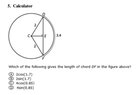# Chord length

• MHB
Gold Member
MHB
View attachment 8904

Ok this should be just an observation solution ..
But isn't the equation for chord length
$$2r\sin{\frac{\theta}{2}}= \textit{chord length}$$

Don't see any of the options
Derived from that..

#### Attachments

•20190315_092610.jpg
7.3 KB · Views: 40

Olinguito
Hi karush.

All the information is there. You just need to calculate the angle $\theta$ from the arc length $s=r\theta$.

Gold Member
MHB
Hi karush.

All the information is there. You just need to calculate the angle $\theta$ from the arc length $s=r\theta$.

It is asking for chord lenght!

Gold Member
MHB
I would start with the arc-length formula to find the subtended angle:

$$\displaystyle \theta=\frac{s}{r}=\frac{3.4}{2}=1.7$$

Then, use the law of cosines:

$$\displaystyle \overline{DF}=\sqrt{2^2+2^2-2(2)(2)\cos(1.7)}=2\sqrt{2-2\cos(1.7)}$$

Lastly, a double-angle identity for cosine:

$$\displaystyle \overline{DF}=2\sqrt{4\sin^2(0.85)}=4\sin(0.85)$$

Gold Member
MHB
Ok
So that's where .85 comes from
So then it's D

Olinguito
It is asking for chord lenght!

And to do that, you need to know the angle $\theta$, don’t you? Calculate that first!

Gold Member
MHB
Why of course we do!

However for this SAT question
We should know that 1.7 is not the $\theta$ we need
and we have use sin $\theta$
That was because you were given arc length $s=3.4$ (and radius $r=2$) but not $\theta$. I was therefore instructing you to compute $\theta$ from the formula $s=r\theta$ so you could use it in the formula $2r\sin\dfrac{\theta}2$ for the chord length.